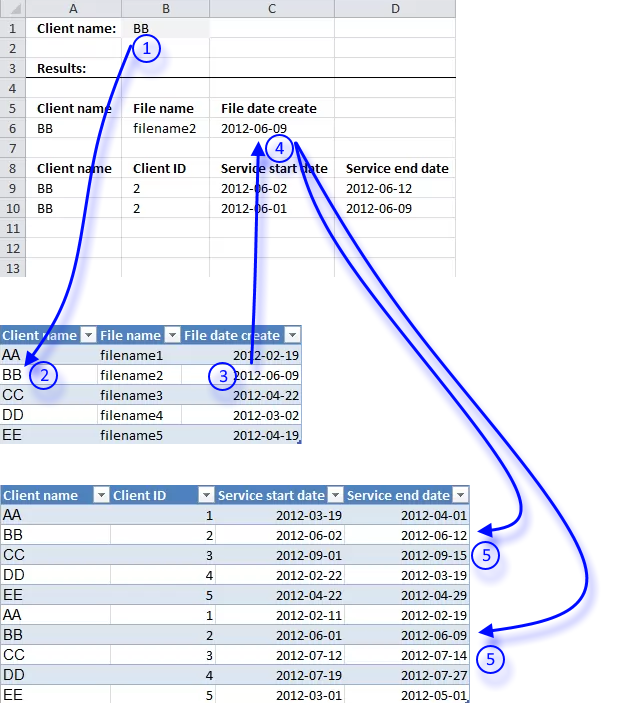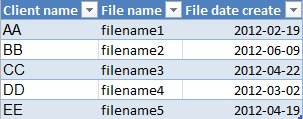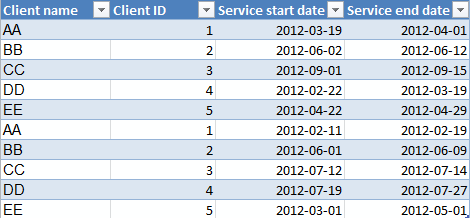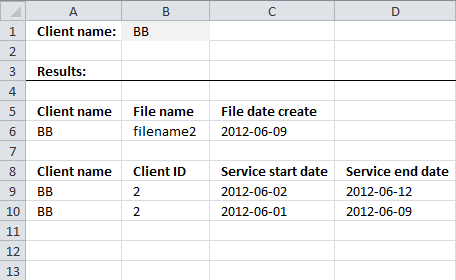Author: Oscar Cronquist Article last updated on October 07, 2019I will in this article demonstrate how to search a table for a date based on a condition and then use that date to search a second table based on the first lookup value and the returned date.

1. The user enters a lookup value in cell B1.
2. A formula finds the value in the first table.
3. Then returns the corresponding date on the same rows as the found lookup value.
4. The date is returned to cell C6.
5. Another formula uses the returned date in cell C6 and the condition in cell B1 to search a second table . It then returns the matching records to cell range A9:D10 if the date is in the date range.

The formula in row 6 is really not necessary, it is only there so you can follow and understand the calculation.

Hi Oscar,I've been trying to find the solution for my lookup problem for a while now and you seem like the right person to ask... Your lookup code works great (thanks) but I need to do two or three lookups within identified matching records... in other words:
Sheet 1 - 'File data'
1. client name
2. filename
3. file date create
Sheet 2 - 'Client data'
1. client name
2. client ID
3. service start date
4. service end date
I need to map correct client ID based on lookup by client name and then based on finding which service date range does client file created date fit into.
So I need to:
1. First search - Identify Client records with matching name
2. Second search - Within that range, I need to find fitting date range.
Your lookups are great when I search entire sheets but I need to do second search based on subset of data.
Any help will be much appreciated.
Thanks!
Nena

I created two tables containing random data.

Sheet1, Table1Sheet2, Table2Sheet 3:Formula in cell A6:

=INDEX(Table1,MATCH(\$B\$1,Table1[Client name],0),COLUMN(A1))

This formula contains a reference to a table. Dragging the cell by the handle to 'pull' the formula across multiple columns won't work, it will mess up the structured references. You have to copy cell A6 and paste to cell range B6:C6.

Array formula in cell A9:

=IFERROR(INDEX(Table2, SMALL(IF((INDEX(Table1[File date create], MATCH(Sheet3!\$B\$1, Table1[Client name], 0))<=Table2[Service end date])*(INDEX(Table1[File date create], MATCH(Sheet3!\$B\$1, Table1[Client name], 0))>=Table2[Service start date])*(\$B\$1=Table2[Client name]), MATCH(ROW(Table2[Client name]), ROW(Table2[Client name])), ""), ROWS(\$A\$1:A1)), COLUMNS(\$A\$1:A1)), "")

#### How to enter an array formula

1. Select cell A9
2. Press with left mouse button on in formula bar3. Paste above array formula
4. Press and hold Ctrl + Shift
5. Press Enter

Copy cell A9 and paste to cell range B9:D9. Copy cell range A9:D9 and paste to cell range A10:D14.

### Explaining formula in cell A9

This formula does not need the calculations returned in row 6 in order to return the correct records.

#### Step 1 - Find the date

The MATCH function allows you to search a column for a specific value, it returns the relative position of the found value. For example, value "BB" is found in the second row so the MATCH function returns 2.

INDEX(Table1[File date create], MATCH(Sheet3!\$B\$1, Table1[Client name], 0))

becomes

INDEX(Table1[File date create], MATCH("BB", Table1[Client name], 0))

becomes

INDEX(Table1[File date create], MATCH("BB", {"AA"; "BB"; "CC"; "DD"; "EE"}, 0))

becomes

INDEX(Table1[File date create], 2)

and returns 6/9/2012.  I recommend that you read How Excel Stores Times if you want to know how Excel handles dates.

#### Step 2 - Check if date is smaller than or equal to the end dates in Table2

The smaller than sign is a logical operator that compares the date to each date in column "Service end date" in Table 2, it returns TRUE or FALSE.

(INDEX(Table1[File date create], MATCH(Sheet3!\$B\$1, Table1[Client name], 0))<=Table2[Service end date])

becomes

(41069<={41000; 41072; 41167; 40987; 41028; 40958; 41069; 41104; 41117; 41030})

and returns {FALSE; TRUE; TRUE; FALSE; FALSE; FALSE; TRUE; TRUE; TRUE; FALSE}.

#### Step 3 - Check if date is larger than or equal to the start dates in Table2

The larger than sign is also a logical operator that compares the found date to each date in column "Service start date" in Table 2, it also returns TRUE or FALSE.

INDEX(Table1[File date create], MATCH(Sheet3!\$B\$1, Table1[Client name], 0))>=Table2[Service start date])

becomes

41069<=Table2[Service start date])

becomes

41069<={40987; 41062; 41153; 40961; 41021; 40950; 41061; 41102; 41109; 40969}

and returns

{FALSE; FALSE; TRUE; FALSE; FALSE; FALSE; FALSE; TRUE; TRUE; FALSE}

#### Step 4 - Check condition

The equal sign lets you compare the value in cell B1 to all values in column "Client name" in Excel Table named Table2.

\$B\$1=Table2[Client name]

becomes

"BB"={"AA"; "BB"; "CC"; "DD"; "EE"; "AA"; "BB"; "CC"; "DD"; "EE"}

and returns

{FALSE; TRUE; FALSE; FALSE; FALSE; FALSE; TRUE; FALSE; FALSE; FALSE}

#### Step 5 - Multiply arrays

All conditions must be TRUE in order to return the correct rows, we can accomplish that by multiplying all arrays.

The parentheses is used to control the order of calculation, we want to perform the comparisons before we multiply the arrays.

(INDEX(Table1[File date create], MATCH(Sheet3!\$B\$1, Table1[Client name], 0))<=Table2[Service end date])*(INDEX(Table1[File date create], MATCH(Sheet3!\$B\$1, Table1[Client name], 0))>=Table2[Service start date])*(\$B\$1=Table2[Client name])

becomes

{FALSE; TRUE; TRUE; FALSE; FALSE; FALSE; TRUE; TRUE; TRUE; FALSE}*{FALSE; FALSE; TRUE; FALSE; FALSE; FALSE; FALSE; TRUE; TRUE; FALSE}*{FALSE; TRUE; FALSE; FALSE; FALSE; FALSE; TRUE; FALSE; FALSE; FALSE}

and returns

{0; 1; 0; 0; 0; 0; 1; 0; 0; 0}.

#### Step 6 - Return the corresponding row number

The IF function replaces the 1's with the corresponding row number and 0's with nothing (blank).

IF((INDEX(Table1[File date create], MATCH(Sheet3!\$B\$1, Table1[Client name], 0))<=Table2[Service end date])*(INDEX(Table1[File date create], MATCH(Sheet3!\$B\$1, Table1[Client name], 0))>=Table2[Service start date])*(\$B\$1=Table2[Client name]), MATCH(ROW(Table2[Client name]), ROW(Table2[Client name])), "")

becomes

IF({0; 1; 0; 0; 0; 0; 1; 0; 0; 0}, MATCH(ROW(Table2[Client name]), ROW(Table2[Client name])), "")

becomes

IF({0; 1; 0; 0; 0; 0; 1; 0; 0; 0}, {1; 2; 3; 4; 5; 6; 7; 8; 9; 10}, "")

and returns

{"";2;"";"";"";"";7;"";"";""}.

#### Step 7 - Calculate k-th smallest row number

The SMALL function has the ability to return the k-th smallest number from a cell range or array.

SMALL(array, k)

SMALL(IF((INDEX(Table1[File date create], MATCH(Sheet3!\$B\$1, Table1[Client name], 0))<=Table2[Service end date])*(INDEX(Table1[File date create], MATCH(Sheet3!\$B\$1, Table1[Client name], 0))>=Table2[Service start date])*(\$B\$1=Table2[Client name]), MATCH(ROW(Table2[Client name]), ROW(Table2[Client name])), ""), ROWS(\$A\$1:A1))

becomes

SMALL({"";2;"";"";"";"";7;"";"";""}, ROWS(\$A\$1:A1))

The ROWS function uses a cell reference that grows automatically when you copy the cell and paste to cells below, this allows the formula to return different values in each cell.

SMALL({"";2;"";"";"";"";7;"";"";""}, ROWS(\$A\$1:A1))

becomes

SMALL({"";2;"";"";"";"";7;"";"";""}, 1)

and returns 2.

#### Step 8 - Return record

The INDEX function lets you fetch a value from a specific cell range based on a row number and a column number.

INDEX(Table2, SMALL(IF((INDEX(Table1[File date create], MATCH(Sheet3!\$B\$1, Table1[Client name], 0))<=Table2[Service end date])*(INDEX(Table1[File date create], MATCH(Sheet3!\$B\$1, Table1[Client name], 0))>=Table2[Service start date])*(\$B\$1=Table2[Client name]), MATCH(ROW(Table2[Client name]), ROW(Table2[Client name])), ""), ROWS(\$A\$1:A1)), COLUMNS(\$A\$1:A1))

becomes

INDEX(Table2, 2, COLUMNS(\$A\$1:A1))

The COLUMNS function uses a cell reference that grows automatically when you copy the cell and paste to cells to the right, this allows the formula to return different values in each cell.

INDEX(Table2, 2, COLUMNS(\$A\$1:A1))

becomes

INDEX(Table2, 2, 1)

and returns "BB" in cell A9.

#### Step 9 - Remove errors

The IFERROR function removes errors when the formula runs out of values, however, be careful with this function. It removes all kinds of formula errors which may make it harder for you to troubleshoot and find errors.

Recommended blog post:
Search two related tables simultaneously (vba)

### Get the Excel fileNena.xlsx